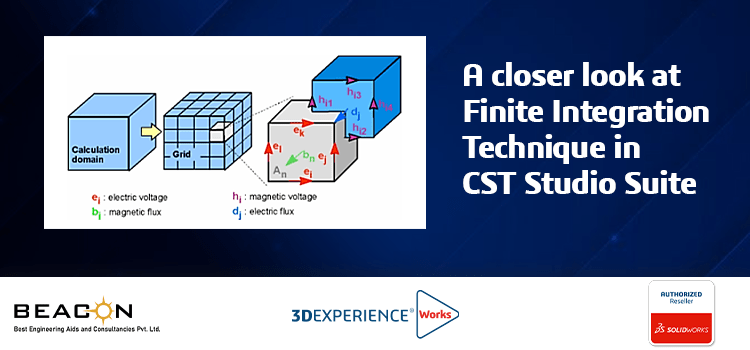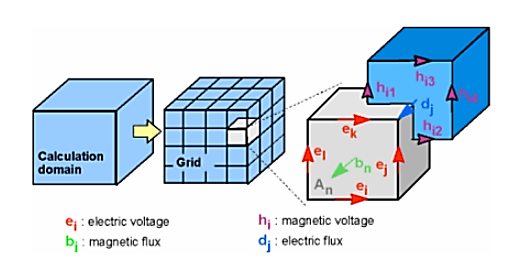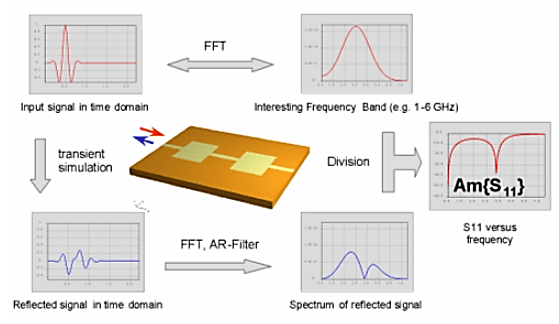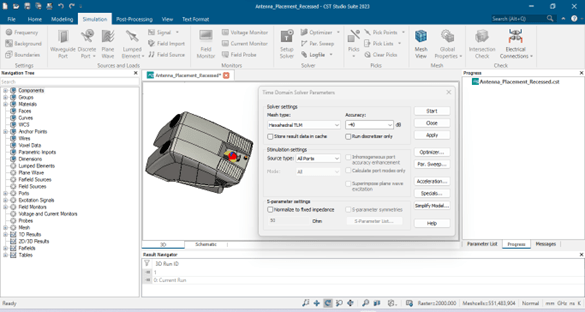# A closer look at Finite Integration Technique in CST Studio SuiteThe Finite Integration Technique (FIT) is a numerical method used in time-domain electromagnetic simulations to solve Maxwell’s equations. This technique is used by the time domain solver in SIMULIA CST Studio Suite, a powerful software package for electromagnetic simulations.

In FIT, Maxwell’s equations are discretized in both space and time. A computational domain is defined, and the domain is discretized using a mesh of cells, and the time is discretized using a time step. The electric and magnetic fields are calculated at each the node of the mesh, and the time evolution of the fields is obtained by integrating Maxwell’s equations over each cell in the mesh.One of the key advantages of FIT is its ability to handle complex geometries. The mesh can be structured or unstructured, allowing for flexibility in modeling a variety of shapes and sizes. Additionally, FIT is capable of handling materials with arbitrary frequency-dependent properties, making it well-suited for simulating a wide range of electromagnetic phenomena.

Another advantage of FIT is its numerical stability. The time step used in FIT must satisfy a stability condition known as the Courant-Friedrichs-Lewy (CFL) condition. This condition ensures that the time step is small enough to accurately capture the high-frequency content of the fields without introducing numerical instabilities. FIT is also a computationally efficient technique. The time evolution of the fields is calculated using a matrix-vector multiplication, which can be efficiently implemented using parallel computing techniques.

For example, let us consider calculating the S-parameters using transient solver. The transient solver operates with time pulses, which can be easily transformed into the frequency domain via a Fast Fourier Transformation (FFT). The S-parameters can then be derived from the resulting frequency domain spectra (Figure 2).In CST Studio Suite, FIT is implemented in the Time Domain Solver module. This module allows users to specify the geometry of the problem, including the materials and boundary conditions, and to set up the simulation parameters, such as the mesh and time step. The module also includes post-processing tools for visualizing the results of the simulation.In conclusion, the Finite Integration Technique is a powerful numerical method used for time-domain electromagnetic simulations. Its ability to handle complex geometries, frequency-dependent materials, and its numerical stability and efficiency make it an ideal choice for a wide range of electromagnetic applications. In CST Studio Suite, FIT is implemented in the Time Domain Solver module, providing users with a comprehensive tool for simulating and analyzing electromagnetic fields in the time domain.

We Urge You To Call Us For Any Doubts & Clarifications That You May Have. We Are Eager to Talk To You

Call Us: +91 7406663589

### Gujarat(No Ratings Yet)Loading...